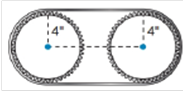Chapter 8.4, Problem 41E### Elementary Geometry for College St...

6th Edition
Daniel C. Alexander + 1 other
ISBN: 9781285195698

#### Solutions

Chapter
Section### Elementary Geometry for College St...

6th Edition
Daniel C. Alexander + 1 other
ISBN: 9781285195698
Textbook Problem
1 views

# In Exercises 34-44, use your calculator value of π unless otherwise stated. Round answers to two decimal places.If two gears, each of radius 4 in are used in a chain drive system with a chain length of 54 in. What is the distance between the centers of the gears.To determine

To Find:

The distance between the centers of two gears

Explanation

The total chain length is 54 in.

The chain semi circularly covers both the gears.

Therefore, the chain forms a circle over the the gears together.

The radius of the gears is 4 in.

The circumference of the circular chain is C=2πr.

Here, π=3.1416.

Therefore, 23.14164=25.13

### Still sussing out bartleby?

Check out a sample textbook solution.

See a sample solution

#### The Solution to Your Study Problems

Bartleby provides explanations to thousands of textbook problems written by our experts, many with advanced degrees!

Get Started

#### Solve for y: 2x+3y=12

Elementary Technical Mathematics

#### Define sampling with replacement and explain why is it used?

Statistics for The Behavioral Sciences (MindTap Course List)

#### In Problems 13-24, find the indicated derivative.

Mathematical Applications for the Management, Life, and Social Sciences

#### Place the following set of n = 20 scores in a frequency distribution table.

Essentials of Statistics for The Behavioral Sciences (MindTap Course List)

#### Simplify each expression. 3

Trigonometry (MindTap Course List)

#### True or False: The graph in question 3 has a vertical asymptote at x = 1.

Study Guide for Stewart's Single Variable Calculus: Early Transcendentals, 8th